16. Tamsin writes down three two-digit integers.

One is square, one is prime and one is triangular. She uses the digits 3,  4,  5,  6,  7 and 8 exactly once each.

Which prime does she write?

A:
B:
C:
D:
E:

17. A rectangle is three times as long as it is high.

The area of a square is twelve times the area of the rectangle.

What is the ratio of the perimeter of the square to the perimeter of the rectangle?

18. What fraction of the integers from 1 to 8000 inclusive are cubes?

A: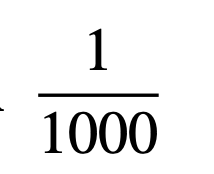B: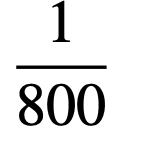C: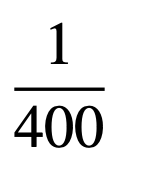D: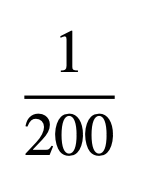E: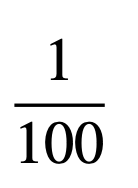19. Each row, each column and each of the bold 2 by 3 rectangles in the grid has to contain each of the numbers 1, 2, 3, 4, 5 and 6

(one number in each cell).

What number should go in the cell marked x?

A:
B:
C:
D:
E:

20. Emily writes down the largest two-digit prime such that each of its digits is prime.

Krish writes down the smallest two-digit prime such that each of its digits is prime.

Kirsten subtracts Krish’s number from Emily’s number.

A:
B:
C:
D:
E:

21. The diagram shows a regular hexagon PQRSTU, a square PQVW and an equilateral triangle V XW.

What is the size of angle XV R?

22. In the multiplication shown alongside, T , R, A and P are all different digits.

What is the value of R?

A:
B:
C:
D:
E:

23. The diagram shows two squares JKLM and PQRS. The length of JK is 6cm and that of PQ is 4 cm. The vertex K is the midpoint of side RS.
What is the area of the shaded region?

24. The diagram shows a regular heptagon.

Which of these expressions is equal to p + q + r + s + t?

Th ediagram shows the first fifteen positive integers arranged in a ‘triangle’.

These numbers are to be rearranged so that the five integers along each ‘edge’ of the triangle have the same sum, unlike the example shown.

When this is done, what is the greatest possible such sum?

A:
B:
C:
D:
E:

Back to the top